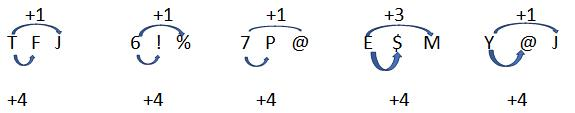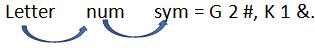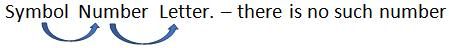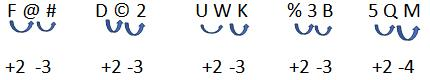Courses

# Test: Letter Series Type - 5

## 15 Questions MCQ Test UPSC CSAT: Logical Reasoning (LR) & Data Interpretation (DI) | Test: Letter Series Type - 5

Description
This mock test of Test: Letter Series Type - 5 for CAT helps you for every CAT entrance exam. This contains 15 Multiple Choice Questions for CAT Test: Letter Series Type - 5 (mcq) to study with solutions a complete question bank. The solved questions answers in this Test: Letter Series Type - 5 quiz give you a good mix of easy questions and tough questions. CAT students definitely take this Test: Letter Series Type - 5 exercise for a better result in the exam. You can find other Test: Letter Series Type - 5 extra questions, long questions & short questions for CAT on EduRev as well by searching above.
QUESTION: 1

### Study the following arrangement and answer the questions below: 5 T J & A F 5 6 % Y J ! 7 @ M # P A 8 + E K A M \$ 4 5 I Which is the 3rd term to the left of the 15th term which is to the left of the 2nd term from the right end?

Solution:

5 T J & A | F 5 6 % Y | J ! 7 @ M | # P A 8 + | E K A M \$ | 4 5 I
We divided series in group of each to save time.
Total elements – 28
2nd from right- 27 its 15 left = 27 – 15 = 12 its 3rd left = 12 – 3 = 9
Which is ‘%’.

QUESTION: 2

### Study the following arrangement and answer the questions below: 5 T J & A F 5 6 % Y J ! 7 @ M # P A 8 + E K A M \$ 4 5 I How many such consonants are there each of which is followed by a vowel but is not preceded by a letter?

Solution:

5 T J & A | F 5 6 % Y | J ! 7 @ M | # P A 8 + | E K A M \$ | 4 5 I
We divided series in group of each to save time.
Consonant followed by vowel but not preceded by letter-
#PA.

QUESTION: 3

### Study the following arrangement and answer the questions below: 5 T J & A F 5 6 % Y J ! 7 @ M # P A 8 + E K A M \$ 4 5 I If all the symbols and vowels are dropped from the series, which will be 10th element from the right end?

Solution:

5 T J & A | F 5 6 % Y | J ! 7 @ M | # P A 8 + | E K A M \$ | 4 5 I
We divided series in group of each to save time.
Series after given condition – 5 T J F 5 6 Y J 7 M P 8 K M 4 5
10th from right –Y.

QUESTION: 4

Study the following arrangement and answer the questions below:
5 T J & A F 5 6 % Y J ! 7 @ M # P A 8 + E K A M \$ 4 5 I

If a vowel gets 1 point, a symbol gets 2 points, all letters that are not vowel get 3 points and the number gets the same number of points that they represent then what is the sum of the series?

Solution:

5 T J & A | F 5 6 % Y | J ! 7 @ M | # P A 8 + | E K A M \$ | 4 5 I
We divided series in group of each to save time.
Sum – 86.

QUESTION: 5

Study the following arrangement and answer the questions below:
5 T J & A F 5 6 % Y J ! 7 @ M # P A 8 + E K A M \$ 4 5 I

Four of the five sequence are alike in some manner. Find the odd one out?

Solution:

5 T J & A | F 5 6 % Y | J ! 7 @ M | # P A 8 + | E K A M \$ | 4 5 I
We divided series in group of each to save time.QUESTION: 6

Study the following arrangement and answer the questions below:
2 R 8 C F 8 E \$ G 2 # 4 9 L C % B < K 1 & A W ? P E = Q @ 7 F 6

If the above sequence is written in reverse order, then which element will be 5th to the right of the 15th element from the right end?

Solution:

2 R 8 C F 8 E \$ | G 2 # 4 9 | L C % B < | K 1 & A W | ? P E = Q | @ 7 F 6
15th from right – 32 + 1 – 15 = 18th from left. Its 5th right = 18 + 5 = 23rd if series reversed – 1 + 32 -23 = 10th. Which is 2.

QUESTION: 7

Study the following arrangement and answer the questions below:
2 R 8 C F 8 E \$ G 2 # 4 9 L C % B < K 1 & A W ? P E = Q @ 7 F 6

How many such numbers are there in the above sequence, each of which is immediately preceded by a constant and immediately followed by a symbol?

Solution:

2 R 8 C F 8 E \$ | G 2 # 4 9 | L C % B < | K 1 & A W | ? P E = Q | @ 7 F 6QUESTION: 8

Study the following arrangement and answer the questions below:
2 R 8 C F 8 E \$ G 2 # 4 9 L C % B < K 1 & A W ? P E = Q @ 7 F 6

Which element is 7th to the left of 16th element from the left in the above sequence?

Solution:

2 R 8 C F 8 E \$ | G 2 # 4 9 | L C % B < | K 1 & A W | ? P E = Q | @ 7 F 6
7th left to 16th from left – 16 – 7 = 9th which is G.

QUESTION: 9

Study the following arrangement and answer the questions below:
2 R 8 C F 8 E \$ G 2 # 4 9 L C % B < K 1 & A W ? P E = Q @ 7 F 6

If first half of the series is written in reverse order, then which element will be 21st from the right end?

Solution:

2 R 8 C F 8 E \$ | G 2 # 4 9 | L C % B < | K 1 & A W | ? P E = Q | @ 7 F 6
21st from right means – second half + 5th from right in first half, that means 5th from left in original series which if – F

QUESTION: 10

Study the following arrangement and answer the questions below:
2 R 8 C F 8 E \$ G 2 # 4 9 L C % B < K 1 & A W ? P E = Q @ 7 F 6

If the second half of the series is written in reverse order, then which element will be 21st from the right end?

Solution:

2 R 8 C F 8 E \$ | G 2 # 4 9 | L C % B < | K 1 & A W | ? P E = Q | @ 7 F 6
21st from right means- second half +5th from right in first half,
That means 12th from left which is 4.

QUESTION: 11

Study the following arrangement and answer the questions below:
E 4 B % R 3 A 6 # F H @ I 2 D 9 © K U \$ W 1 M P 5 * Q 8 T

If all the numbers are dropped from the above arrangement, Which one of the following will be the 9th to the left of W?

Solution:

E 4 B % R 3 A 6 | # F H @ I | 2 D 9 © K | U \$ W 1 M | P 5 * Q 8 T |
W = 21st , its 9th left = 21 - 9 = 12, numbers in between = 2,
Therefore element is F.

QUESTION: 12

Study the following arrangement and answer the questions below:
E 4 B % R 3 A 6 # F H @ I 2 D 9 © K U \$ W 1 M P 5 * Q 8 T

How many such numbers are there in the above arrangement each of which is immediately preceded by a symbol and immediately followed by a letter?

Solution:

E 4 B % R 3 A 6 | # F H @ I | 2 D 9 © K | U \$ W 1 M | P 5 * Q 8 T |QUESTION: 13

Study the following arrangement and answer the questions below:
E 4 B % R 3 A 6 # F H @ I 2 D 9 © K U \$ W 1 M P 5 * Q 8 T

Which of the following is 5th to the right of 18th from the right?

Solution:

E 4 B % R 3 A 6 | # F H @ I | 2 D 9 © K | U \$ W 1 M | P 5 * Q 8 T |
18th from right = 29 + 1 – 18 = 12th from left, its 5th right = 12 + 5 = 17th = ©.

QUESTION: 14

Study the following arrangement and answer the questions below:
E 4 B % R 3 A 6 # F H @ I 2 D 9 © K U \$ W 1 M P 5 * Q 8 T

Which of the following is 4th to the right of 12th from the left end?

Solution:

4 B % R 3 A 6 | # F H @ I | 2 D 9 © K | U \$ W 1 M | P 5 * Q 8 T |
4th right to 12th from left = 12 + 4 = 16th = 9.

QUESTION: 15

Study the following arrangement and answer the questions below:
E 4 B % R 3 A 6 # F H @ I 2 D 9 © K U \$ W 1 M P 5 * Q 8 T

Four of the below five are alike in a certain way. Find the odd one?

Solution:

4 B % R 3 A 6 | # F H @ I | 2 D 9 © K | U \$ W 1 M | P 5 * Q 8 T |